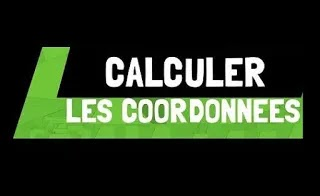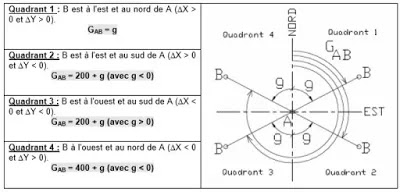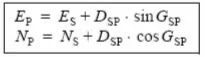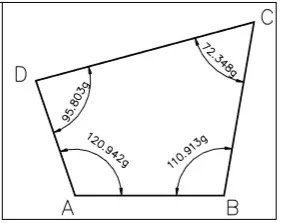How to calculate the coordinates of a vector ₪ 2.2 Calculation of Cartesian coordinates from a bearing

# How to calculate the coordinates of a vector ₪ 2.2 Calculation of Cartesian coordinates from a bearing### How to calculate the mathematicl coordinates of a point in a coordinate system and the mathematical coordinates of a vector.

Calculation of topographic coordinates
The  horizontal circle (or limb) is the graduation of the theodolite on which the operator reads the horizontal angles. It is linked to the base of the device but can also pivot on itself so as to adjust the zero of the graduations in a given direction.
The graduations are increasing from 0 tgo 400 gon clockwise.
After the theodolite  has been set up, this circle is horizontal plane and called horizontal (or azimuth)angles, denoted Hz. :1.2 Terminology of horizontal angle mzasurementsThe device being in its reference position and the zero of the horizontal scale not being modified after stationing, the operator takes an azimuthal reading LA on point A then an LB reading on B and deduces the angle ASB:Definition :The bearing of a direction AB is the horizontal angle measured positively in the clockwise direction between the ordinate axis of the projection system used and this direction AB .
It is denoted GAB Mathematically, it is the positive an gle in the clockwise direction between the ordinateb axis of the reference and the line (AB).
A bearing is always between 0 and 400 geo. GAB is the angle betwee, the North (ordinates) and the direction AB
GBA is the angle between the North and the BA direction. The relartionship between GAB and GBA is :2. Uses of deposits
2.1 Calculation of a bearing from Cartesian coordinates

Consider the coordinates of two points A (EA, NA) and B (EB, NB BB)
The following relationship is used to calculate GAB :Exercise n°1 :
Calculate from the formula the bearing of the following direction AB with:
A (10 ; 50) and B (60 ; 10) the lines SA whose lenght Dp the operator has measured. It reads the corresponding angle V, it measures Dp and deduces that :2.2 Calculation of Cartesian coordinates from a bearing
In topography, it is very common to know a point S (ES , NS) and to seek the coordinates of a point P visible from S .
We say that P is radiated from S if we can measure the horizontal distance DSP znf thr bearing GSP. Whatever the quadrant, we can then calculate the coordinates of the point P by the following formulas :Exercise n°2 :
S (680 379,84 ; 210 257,06) is given in Lambert coorfinate (m), calculate the coorinates of P such that : DSP = 45,53 m and GSP = 172,622 gon.

3. Calculation and compensation of polygons
3.1 Definition

A polygon is a set of linked survey lines, each of which has its length and the angles defined between them. A polygon is necessarily closed to itself and can have any number of vertices. The angles at each of the vertices can be interior or exterior angles.3.2  Close gap and angular compensations

Returning to the exemple of the polygon above, we can establish the angular closure defined by the field measurements. To calculate an angular closure deviation, the difference is made between the raw sum of the measurement and the sum that the angles of a polygon of 'n' sides should theoretically make, ie 200 gon x (n-2).